# 9.3 Summary

The following theorem summarizes the performance of the RedBlackTree data structure:

Theorem 9..1   A RedBlackTree implements the SSet interface and supports the operations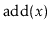,, and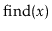inworst-case time per operation.

Not included in the above theorem is the following extra bonus:

Theorem 9..2   Beginning with an empty RedBlackTree, any sequence of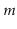and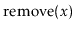operations results in a total of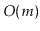time spent during all calls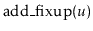and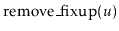.

We only sketch a proof of Theorem 9.2. By comparingandwith the algorithms for adding or removing a leaf in a 2-4 tree, we can convince ourselves that this property is inherited from a 2-4 tree. In particular, if we can show that the total time spent splitting, merging, and borrowing in a 2-4 tree is, then this implies Theorem 9.2.

The proof of this theorem for 2-4 trees uses the potential method of amortized analysis.9.2 Define the potential of an internal node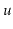in a 2-4 tree as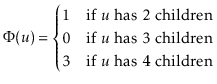and the potential of a 2-4 tree as the sum of the potentials of its nodes. When a split occurs, it is because a node with four children becomes two nodes, with two and three children. This means that the overall potential drops by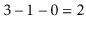. When a merge occurs, two nodes that used to have two children are replaced by one node with three children. The result is a drop in potential of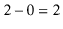. Therefore, for every split or merge, the potential decreases by two.

Next notice that, if we ignore splitting and merging of nodes, there are only a constant number of nodes whose number of children is changed by the addition or removal of a leaf. When adding a node, one node has its number of children increase by one, increasing the potential by at most three. During the removal of a leaf, one node has its number of children decrease by one, increasing the potential by at most one, and two nodes may be involved in a borrowing operation, increasing their total potential by at most one.

To summarize, each merge and split causes the potential to drop by at least two. Ignoring merging and splitting, each addition or removal causes the potential to rise by at most three, and the potential is always non-negative. Therefore, the number of splits and merges caused byadditions or removals on an initially empty tree is at most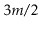. Theorem 9.2 is a consequence of this analysis and the correspondence between 2-4 trees and red-black trees.

#### Footnotes

... analysis.9.2
See the proofs of Lemma 2.2 and Lemma 3.1 for other applications of the potential method.
opendatastructures.org